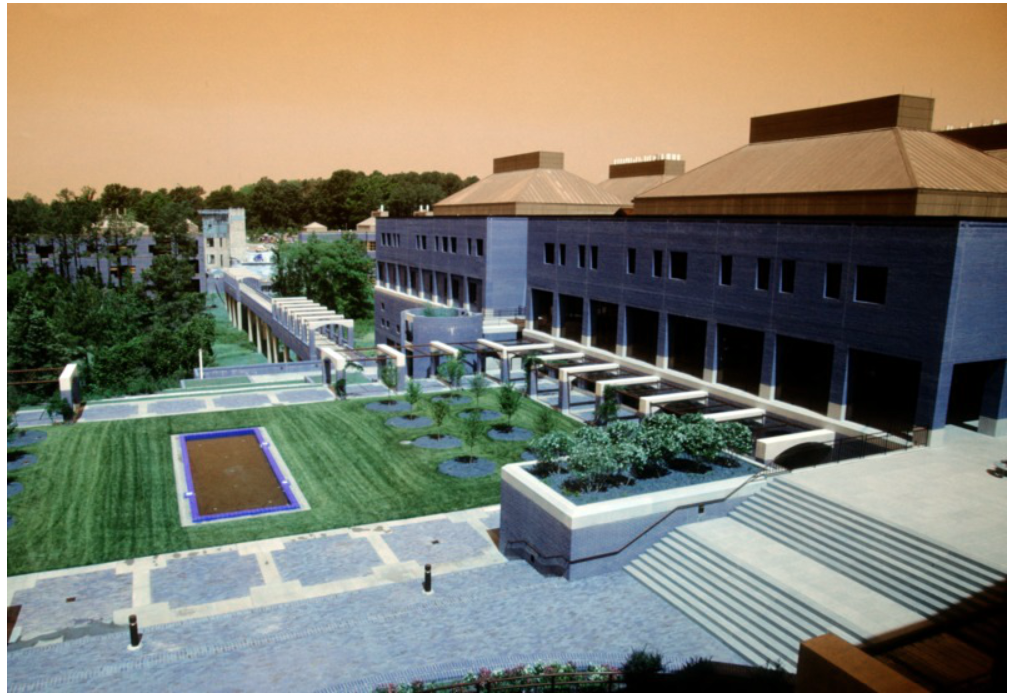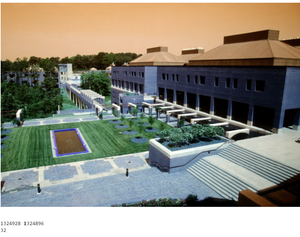Open in App
Not now

# Impact of Image Flattening

• Difficulty Level : Medium
• Last Updated : 22 Jan, 2021

Flattening is a technique that is used to convert multi-dimensional arrays into a 1-D array, it is generally used in Deep Learning while feeding the 1-D array information to the classification model.

What is the need for Flattening of an Image?

Multi-Dimensional arrays take more amount of memory while 1-D arrays take less memory, which is the most important reason why we flatten the Image Array before processing/feeding the information to our model. In most cases, we will be dealing with a dataset which contains a large amount of images thus flattening helps in decreasing the memory as well as reducing the time to train the model.

Step 1: Importing the necessary libraries

## Python3

 `import` `numpy as np``import` `pandas as pd``import` `cv2 as cv``from` `google.colab.patches ``import` `cv2_imshow``from` `skimage ``import` `io``from` `PIL ``import` `Image``import` `matplotlib.pylab as plt``from` `numpy ``import` `array``from` `sys ``import` `getsizeof`

Step 2: Fetching a random image through web

## Python3

 `#Fetching the url and showing the image using cv2_imshow``urls``=``[``"https://iiif.lib.ncsu.edu/iiif/0052574/full/800,/0/default.jpg"``]``for` `url ``in` `urls:``  ``image ``=` `io.imread(url)``  ``cv2_imshow(image)``  ``print``(``'\n'``)`Step 3: Transforming the image into a multi-dimensional array

## Python3

 `#Getting the multi-dimensional array from the image``array1 ``=` `array(image)``#Memory occupied by the multi-dimensional array``size1 ``=` `getsizeof(array1)``print``(array1)`

Step 4: Now Flattening the multi-dimensional array using flatten() function

## Python3

 `#Using Flatten function on array 1 to convert the multi-dimensional ``# array to 1-D array``array2 ``=` `array1.flatten()``#Memory occupied by array 2``size2 ``=` `getsizeof(array2)``#displaying the 1-D array``print``(array2)`

Step5: Results of Flattening

## Python3

 `#Print's the two different size's of the array``print``(f``"Size of Multidimensional Image : {size1}"``)``print``(f``"Size of Flattened Image : {size2}"``)``difference ``=` `size1 ``-` `size2``#Print's the difference of memory between the size of Multidimensional & 1-D array``print``(``"Size difference in the images: "``, difference)`

```Size of Multidimensional Image : 1324928
Size of Flattened Image : 1324896
Size difference in the images: 32```

Step 6: Full Code

## Python3

 `#importing libraries``import` `numpy as np``import` `pandas as pd``import` `cv2 as cv``from` `google.colab.patches ``import` `cv2_imshow``from` `skimage ``import` `io``from` `PIL ``import` `Image``import` `matplotlib.pylab as plt``from` `numpy ``import` `array``from` `sys ``import` `getsizeof`` ` `#Fetching the url and showing the image using cv2_imshow``urls``=``[``"https://iiif.lib.ncsu.edu/iiif/0052574/full/800,/0/default.jpg"``]``for` `url ``in` `urls:``  ``image ``=` `io.imread(url)``  ``cv2_imshow(image)``  ``print``(``'\n'``)`` ` `#Getting the multi-dimensional array from the image``array1 ``=` `array(image)``#Memory occupied by the multi-dimensional array``size1 ``=` `getsizeof(array1)``print``(array1)`` ` `#Using Flatten function on array 1 to convert the multi-dimensional ``# array to 1-D array``array2 ``=` `array1.flatten()``#Memory occupied by array 2``size2 ``=` `getsizeof(array2)``#displaying the 1-D array``print``(array2)`` ` `#Print's the two different size's of the array``print``(f``"Size of Multidimensional Image : {size1}"``)``print``(f``"Size of Flattened Image : {size2}"``)``difference ``=` `size1 ``-` `size2``#Print's the difference of memory between the size of Multidimensional & 1-D array``print``(difference)`Conclusion:

After running the whole code we see that there is not a major difference in memory used in the multi-dimensional image array and the flattened array. Then people may ask why we are doing the flattening when the effect is negligible. In a large datasets when we are dealing with thousands of images the net amount of the memory saved due to all the images accumulates to be pretty big.

My Personal Notes arrow_drop_up